NCERT Solutions for Class 6 Maths Chapter 12 Ratio and Proportion Ex 12.2 are part of NCERT Solutions for Class 6 Maths. Here we have given NCERT Solutions for Class 6 Maths Chapter 12 Ratio and Proportion Ex 12.2.

 Board CBSE Textbook NCERT Class Class 6 Subject Maths Chapter Chapter 12 Chapter Name Ratio and Proportion Exercise Ex 12.2 Number of Questions Solved 4 Category NCERT Solutions

## NCERT Solutions for Class 6 Maths Chapter 12 Ratio and Proportion Ex 12.2

Question 1.
Determine if the following are in proportion:
(a) 15, 45, 40, 120
(b) 33, 121, 9, 96
(c) 24, 28, 36, 48
(d) 32, 48, 70, 210
(e) 4, 6, 8, 12
(f) 33, 44, 75, 100
Solution :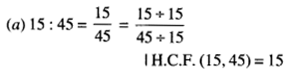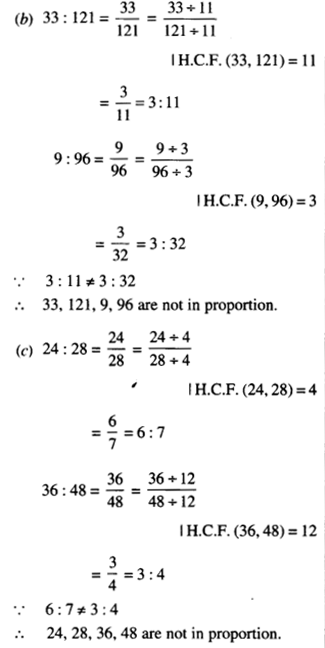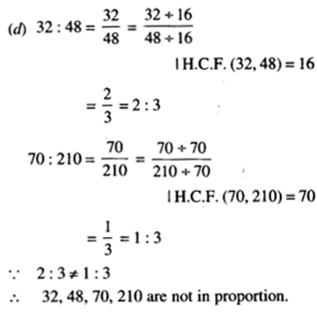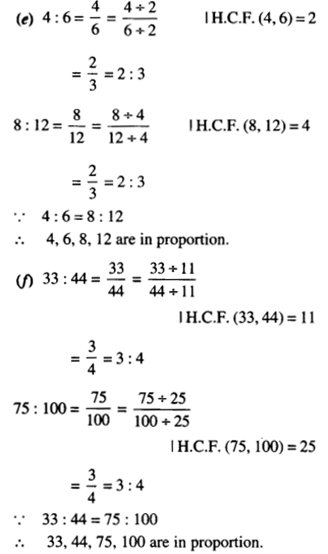Question 2.
Write True (T) or False (F) against each of the following statements :
(a) 16: 24: : 20: 30
(b) 21: 6: 35: 10
(c) 12: 18: 28: 12
(d) 8: 9: : 24: 27
(e) 5.2: 3.9: : 3: 4
(f) 0.9: 0.36: : 10: 4
Solution :Question 3.
Are the following statements true₹ I
(a) 40 Persons : 200 Persons =₹ 15: ₹75 ;
(b) 7.5 litre : 15 litre = 5 kg : 10 kg 1
(c) 99 kg : 45 kg = f 44 : ₹20
(d) 32 m : 64 m = 6 sec: 12 sec
(e) 45 km: 60 km = 12 hours : 15 hours.
Solution :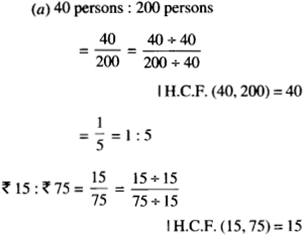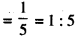Since the two ratios are equal, therefore, the given statement is true.Since the two ratios are equal, therefore, the given statement is true.Since, the two ratios are equal, therefore, the given statement is true.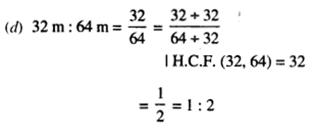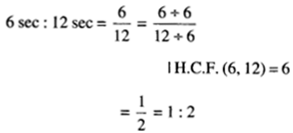Since the two ratios are equal, therefore the given statement is true.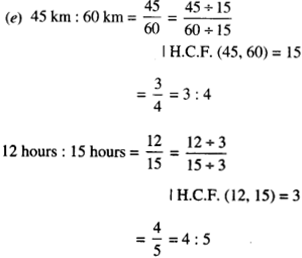Since the two ratios are not equal, therefore the given statement is false.

Question 4.
Determine if the following ratios form a proportion. Also, write the middle terms and extreme terms where the ratios form a proportion.
(a) 25 cm: 1 m and 7 40 : 7160
(b) 39 litre : 65 litre and 6 bottle : 10 bottle
(c) 2 kg : 80 kg and 25 g : 625 g
(d) 200 ml: 2.5 litre and ₹4 ; ₹ 50.
Solution :
(a)
∴ 1 m= 100 cmSince the two ratios are equal, therefore, the given ratios are in proportion. Middle terms are 1 m and ₹ 40. Extreme terms are 25 cm and ₹ 160.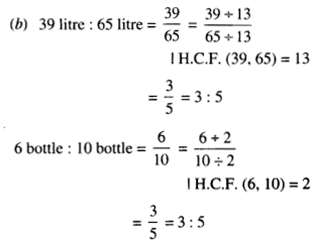Since the two ratios are equal, therefore, the given ratios are in proportion. Middle terms are 65 liters and 6 bottles. Extreme terms are 39 liters and 10 bottles.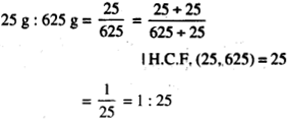Since the two ratios are not equal, therefore, the given ratios are not in proportion.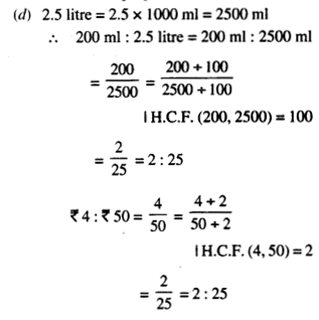Since the two ratios are equal, therefore, the given ratios are in proportion. Middle terms are 2.5 litres and ₹ 4. Extreme terms are 200 ml and ₹ 50.

We hope the NCERT Solutions for Class 6 Maths Chapter 12 Ratio and Proportion Ex 12.2 help you. If you have any query regarding NCERT Solutions for Class 6 Maths Chapter 12 Ratio and Proportion Ex 12.2, drop a comment below and we will get back to you at the earliest.